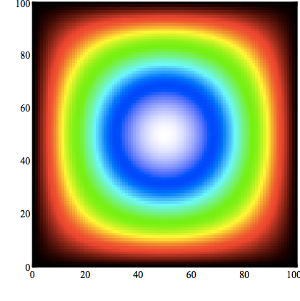# fjsousa/poisson-solver

Node Implementation of a finite diference poisson solver
JavaScriptFetching latest commit…
Cannot retrieve the latest commit at this time.
Type Name Latest commit message Commit time
Failed to load latest commit information.docstest.gitignoreREADME.mdexample.jspackage.jsonpoisson-browser.jspoisson.js

# poisson-solver

Javascript Implementation of a finite differences poisson solver.

##Getting started

```var Poisson = require('.');

//Set conditions
var conditions = {
w: 1,		//width or x dimension
h: 1,		//height or y dimension
n: 50, 	//number of points in the y dimension
m: 50		//number of points in the x dimension
};

//Initialize solver
var poisson = new Poisson(conditions);

var N = [];
var S = [];
var E = [];
var W = [];

for (var i = 0; i < conditions.n; i++) {
N[i] = S[i] = E[i] = W[i] = 0;
}

//Set Dirichlet boundary conditions to zero
poisson.setBoundaryConditions(N, S, E, W);

//Maximum number of iterations allowed
var maxItterations = 100000000;

//Maximum residue allowed
var maxResidue = 1E-9 ;

//Run solver
var output = poisson.solver( maxItterations, maxResidue);

console.log('Solver converged with', output.iterations, 'iterations and', output.residue, 'residue.');

//Print solution
poisson.print('./field.txt', poisson.u.old);

//Print analytical solution
poisson.analytical();
poisson.print('./analytical.txt', poisson.u.analytical);
```

You can visualize the field with your favorite plotting software. I would recommend veusz. Here's how it should look:##Browser

If you have browserify installed you can create a browser version of the poisson solver. Just run:

`npm run browserify`

and then include a script tag on your html file:

`<script type='text/javascript', src='poisson-browser.js'></script>`

Now you can require and use the solver in the browser like you would do with node:

`var Poisson = require('poisson');`
You can’t perform that action at this time.